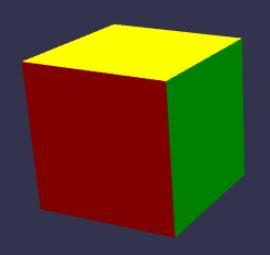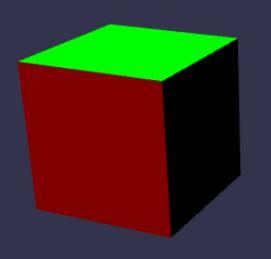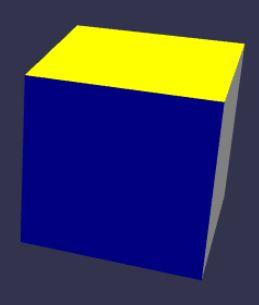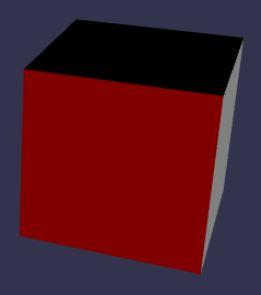# Quaternion to euler angles

## Recommended Posts

Hello Babylon community,

I have troubles to understand how to switch from quaternion to euler angles and vice versa.

First problem:

When I apply a rotation to a cube on axe X which should be a 90 degrees (when the face facing us get on the top) I get a value above 130 degrees from my convertion (quaternion to euler).

Second problem:

When I go over 140 degree on axe X, axes Y and Z are changed! However I'm just playing with X axe.

Am I missing something?

Here a playground: https://playground.babylonjs.com/#7QNRN2#1

Press the key 'a' to rotate on the axe X and watch the console to see the euler angles convert from radian to degrees.

Keys mapping:

• keys 'a' and 'd' for rotation on X
• keys 'w' and 's' for rotation on Y
• keys 'q' and 'e' for rotation on Z
##### Share on other sites

You have probably already read this but in case you have not http://doc.babylonjs.com/resources/rotation_conventions

##### Share on other sites

Yes I did read this article but I still don't understand why when you rotate above 90 degrees on axe X all axis are changed.

I did a mistake in code when converting radian to degrees which fix problem 1.

So for my second problem if I apply a rotation of 91 degrees like below :

``````mesh.rotationQuaternion = BABYLON.Quaternion.RotationYawPitchRoll(
0,
(91 * Math.PI) / 180, // 91 degrees convert to rad
0
);
var euler = quaternion.toEulerAngles();
``````

I get thi following results:

• euler.x = 89 degrees, but should be 91
• euler.y = 180 degrees, but should be 0
• euler.z = 180 degrees, but should be 0

Here an updated version of my playground: https://playground.babylonjs.com/#7QNRN2#2

press key 'a' gonna rotate 91 degrees on axe X and show the results in the console

##### Share on other sites

And for more informations if I do same on the others axis.

So if I rotate 91 degrees  or whatever on axis Y and Z.

I do get back the good results from quaternion.toEulerAngles()

##### Share on other sites

Hi @Seba and welcome to the forum from me.

11 hours ago, Seba said:

I still don't understand why when you rotate above 90 degrees on axe X all axis are changed﻿.

It is because converting from quaternion to Euler Angles is not done using a 1 to 1 mapping.

Take the simple equation y = x2 , this is not 1 to 1 since both 2 and -2 lead to the same result, the function Math.sqrt(4) has to be written to give a result and the convention calculate and return the positive square root is used.

It is the same with rotations.

Take this cube ( all rotations given in degrees)and rotate using the Euler Angles (0, 0, 90) to giveStarting with the same cuberotate using the Euler Angles (180, 180, 270)

first rotate 180 about the local Y axisfollowed by a rotation of 180 about the local X axis to giveand finally a rotation of 270 about the local Z axis to giveSo the Euler angles (0, 0, 90) and (180, 180, 270) give the same orientation to the box.

Reversing this says given the that this boxwas rotated to this orientationwhat are the Euler angles that produce this orientation? As there are more than one answer any algorithm to find the Euler angles must use some convention to produce a result.

As you can see from this playground http://www.babylonjs-playground.com/#1ST43U#74

your Euler angles of (91, 0, 0) and (89, 180, 180) produce the same orientation and it just happens that the convention used by the toEulerAngles function produces the second result.

Hope all is now explained.

##### Share on other sites

Ok got it!

Thanks a lot for this clear explanation.

## Join the conversation

You can post now and register later. If you have an account, sign in now to post with your account.
Note: Your post will require moderator approval before it will be visible.×   Pasted as rich text.   Paste as plain text instead

Only 75 emoji are allowed.